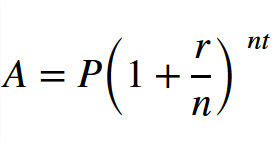# What Is Compounding? Compound Interest Calculator, Formula & Investments

## Formula to Calculate Compound Interest

The mathematical formula for compound interest reads as follows:As text, it reads like this:

FV = P(1 + r/n)(nt)

Where:

• FV = Future Value: The future amount you’ll end up with, including interest
• P = Principal: Your initial investment amount
• r = Rate of Return: The annual rate of return (or interest), as a decimal
• n = Compounding Frequency: The number of times that returns are compounded per year (e.g. 12 times per year means compounded monthly)
• t = Time Period: The number of years you leave the money invested

### Calculate Compound Interest Example

Got all that? I know, my high school math is rusty too. All right, let’s run an example.

You invest \$1,000 of initial principal at 9% interest for two years, compounded monthly. The formula looks like this:

\$1,196.41 = \$1,000(1 + 0.09/12)(12×2)

If you left the money invested for a five year period of time instead, the formula would look like this:

\$1,565.68 = \$1,000(1 + 0.09/12)(12×5)

Or, if you earned a 15% return over five years, the formula to calculate compound interest looks like:

\$2,107.18 = \$1,000(1 + 0.15/12)(12×5)

### Formula for Compound Interest in Excel

To run this formula in an Excel spreadsheet, it looks like this:

=P*(1+(r/n)^(n*t)

To use the latest example from above, with a 15% return compounded monthly for five years, the Excel formula would read like this:

=1000*(1+0.15/12)^(12*5)

That said, Excel actually has a built-in formula for calculating compound interest. It works like this:

=FV((r/n),(n*t),pmt,-PV)

Note that “pmt” refers to additional periodic payment withdrawals, which we’re ignoring for now. So, the Excel formula for the 15% interest investment over five years, compounded monthly, looks like this:

=FV((0.15/12),60,0,-1000)

### Formula for Compound Interest with Monthly Contributions

Ready for the math to get more complicated?

Here’s what the formula looks like to calculate compound interest with monthly investments:In text, the compound interest formula looks like this:

FV = P(1 + r/n)(nt) + PMT((1 + r/n)(nt))/(r/n)

You’d be shocked at how many websites get this formula for additional deposits wrong, by the way. But I digress.

Have fun plugging away at that compound interest formula. I don’t plan on ever using it again, after blowing hours building that dang online calculator.

## Investments with Compound Interest

What investments generate compound returns?

Technically, any investment that generates income can create compound returns, if you reinvest the income back into more investments.

Still, some investments lend themselves better to compound interest than others. Here are a few of my favorite investments for compound interest.

### Rental Properties

You knew this one would make an appearance.

If you reinvest your real estate cash flow into more real estate investments, you start earning returns on your returns. Look no further than the example above, where you put your rents aside and use them to buy more properties. With each property you buy, you earn more monthly cash flow, and can buy the next property even faster.

The trick, of course, is not to touch your profits, but to reinvest them.

For a clever way to invest with none of your own money tied up in each property, read up on the BRRRR method. In fact, rental properties help you bend the 4% Rule in retirement planning, with their ongoing passive income and your ability to invest with leverage.

### House Flipping

Say you flip a house with \$50,000 of your own money as a down payment and for closing costs. After renovating, you sell the property for a \$50,000 profit.

You now have \$100,000 in investment capital, so next time you flip a higher-end property with twice the profit potential. After completing the renovations, you sell the house for a \$100,000 profit.

With \$200,000 in investment capital, you buy two properties to flip next time around. And so it goes, as you exponentially grow your capital.

For a fun read, check out these ideas to flip a house with no money down.

### Real Estate Crowdfunding Investments

You already saw the example above with Streitwise, which pays 8.4% annual dividends. If you reinvest those dividends every quarter, your initial investment compounds on itself.

But Streitwise is far from the only example of a good real estate crowdfunding investment for compound returns. Fundrise pays between 3-5% in dividends each year, on top of hefty appreciation. You can opt to reinvest those dividends automatically, just like Streitwise.

Another great investment for compound interest is Groundfloor. They issue short-term loans paying annual interest rates from 6-15%, which you can reinvest automatically once the borrower repays the loan in full.

YieldStreet paid an 8% dividend in 2021, which you can reinvest automatically. Concreit pays a more modest 5.5% dividend, but lets you withdraw your money at any time. RealtyMogul offers two different REITs, one with a 4.5% dividend and the other paying a 6% dividend. And so it goes.

Reinvest the dividends and reap the rewards of compounded returns.